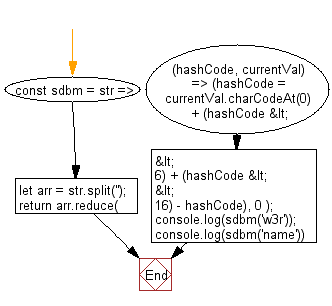# JavaScript : Hash an given input string into a whole number

## JavaScript fundamental (ES6 Syntax): Exercise-99 with Solution

Write a JavaScript program to hash an given input string into a whole number.

Hashes the input string into a whole number.

• Use String.prototype.split('') and Array.prototype.reduce() to create a hash of the input string, utilizing bit shifting.

Sample Solution:

JavaScript Code:

``````//#Source https://bit.ly/2neWfJ2
const sdbm = str => {
let arr = str.split('');
return arr.reduce(
(hashCode, currentVal) =>
(hashCode = currentVal.charCodeAt(0) + (hashCode << 6) + (hashCode << 16) - hashCode),
0
);
};
console.log(sdbm('w3r'));
console.log(sdbm('name'));
```
```

Sample Output:

```986464758
-3521204949
```

Flowchart:Live Demo:

See the Pen javascript-basic-exercise-99-1 by w3resource (@w3resource) on CodePen.

Improve this sample solution and post your code through Disqus

What is the difficulty level of this exercise?

Test your Programming skills with w3resource's quiz.

﻿

## JavaScript: Tips of the Day

Chunks an array into n smaller arrays

Example:

```const tips_chunkIntoN = (arr, n) => {
const size = Math.ceil(arr.length / n);
return Array.from({ length: n }, (v, i) =>
arr.slice(i * size, i * size + size)
);
}
console.log(tips_chunkIntoN([1, 2, 3, 4, 5, 6, 7,8], 4));
```

Output:

```[[1,2],[3,4],[5,6],[7,8]]
```